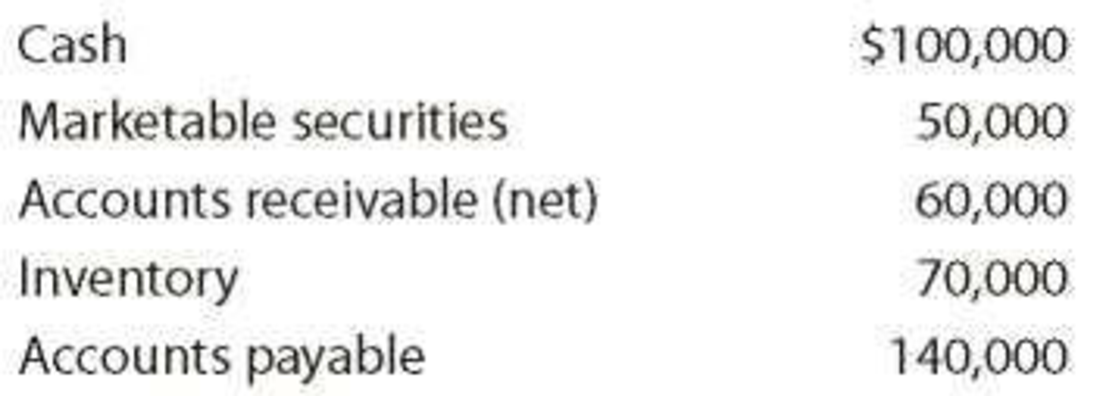# Current position analysis The following items are reported on a company’s balance sheet: Determine (a) the current ratio and (b) the quick ratio. Round to one decimal place.### Financial Accounting

15th Edition
Carl Warren + 2 others
Publisher: Cengage Learning
ISBN: 9781337272124

#### Solutions

Chapter
Section### Financial Accounting

15th Edition
Carl Warren + 2 others
Publisher: Cengage Learning
ISBN: 9781337272124
Chapter 17, Problem 3PEA
Textbook Problem
1 views

## Current position analysisThe following items are reported on a company’s balance sheet:Determine (a) the current ratio and (b) the quick ratio. Round to one decimal place.

a)

To determine

Compute Current ratio.

### Explanation of Solution

Financial Ratios: Financial ratios are the metrics used to evaluate the liquidity, capabilities, profitability, and overall performance of a company.

Current Ratio: Current ratio is used to determine the relationship between current assets and current liabilities. Current ratio is determined by dividing current assets and current liabilities.

Formula:

Current ratio=Current assetsCurrentliabilities

The ideal current ratio is 2:1

Compute current ratio.

Current ratio=Current assetsCurrent liabilities=$280,000$140,000=2

b)

To determine

Calculate Acid-test ratio.

### Still sussing out bartleby?

Check out a sample textbook solution.

See a sample solution

#### The Solution to Your Study Problems

Bartleby provides explanations to thousands of textbook problems written by our experts, many with advanced degrees!

Get Started

Find more solutions based on key concepts
Give three examples of important trade-offs that you face in your life.

Principles of Microeconomics (MindTap Course List)

Why are budget deficits and trade deficits sometimes called the twin deficits?

Brief Principles of Macroeconomics (MindTap Course List)

Why is the journal called a book of original entry?

College Accounting (Book Only): A Career Approach

PREFERRED STOCK RATE OF RETURN What will be the nominal rate of return on a perpetual preferred stock with a 10...

Fundamentals of Financial Management, Concise Edition (with Thomson ONE - Business School Edition, 1 term (6 months) Printed Access Card) (MindTap Course List)

What are the principal tax implications that should be considered in Year 0?

Cornerstones of Cost Management (Cornerstones Series)